## ISC Chemistry Previous Year Question Paper 2018 Solved for Class 12

Maximum Marks: 70
Time allowed: 3 hours

• Candidates are allowed additional 15 minutes for only reading the paper.
• They must NOT start writing during this time.
• All questions are compulsory.
• Question 1 is of 20 marks having four subparts, all of which are compulsory.
• Question numbers 2 to 8 carry 2 marks each, with two questions having an internal choice.
• Question numbers 9 to 15 carry 3 marks each, with two questions having an internal choice.
• Question numbers 16 to 18 carry 5 marks each, with an internal choice.
• All working, including rough work, should be done on the same sheet as, and adjacent to the rest of the answer.
• The intended marks for questions or parts of questions are given in brackets [ ].
• Balanced equations must be given wherever possible and diagrams where they are helpful.
• When solving numerical problems, all essential working must be shown.
• In working out problems, use the following data:
• Gas constant R = 1.987 cal deg-1 mol-1 = 8.314 JK-1 mol-1 = 0.0821 dm3 atm K-1 mol-1
• 11 atm = 1 dm3 atm = 101.3 J. 1 Faraday = 96500 coulombs.
• Avogadro’s number = 6.023 × 1023.

Question 1.
(a) Fill in the blanks by choosing the appropriate word/words from those given in the brackets: [4 × 1]
(square pyramidal, electrical, 74, 26, sp3d2, sp3d, chemical, 68, 32, tetrahedral, yellow, white, iodoform, Lucas)
(i) A Galvanic cell converts …….. energy into ………… energy.
(ii) The percentage of unoccupied spaces in bcc and fee arrangements are ……….. and …….. respectively.
(iii) Propan-2-ol on reaction with iodine and sodium hydroxide gives ……….. precipitate and the reaction is called ………. test.
(iv) The geometry of XeOF4 molecule is ………… and the hybridisation of xenon atom in the molecule is

(b) Complete the following statements by selecting the correct alternative from the choices given: [4 × 1]
(i) During the course of an SN1 reaction, the intermediate species formed is:
(1) a carbocation
(3) a carbarn on
(4) an intermediate complex

(ii) Purification of aluminium by electrolytic refining is called:
(1) Speck’s process
(2) Hoppe’s process
(3) Hall’s process
(4) Baeyer’s process

(iii) An aqueous solution of urea freezes at -0.186°C, Kf for water = 1.86 K kg mol-1, Kb for water = 0.512 K kg mol-1. The boiling point of the urea solution will be:
(1) 373.065 K
(2) 373.186 K
(3) 373.512 K
(4) 373.0512 K

(iv) In the dehydration of alcohols to alkenes by heating with concentrated sulphuric acid, the initiation step is:
(1) formation of carbocation
(2) formation of an ester
(3) protonation of the alcohol molecule
(4) elimination of water

(c) Match the following: [4 × 1]

 (i) Rate constant (a) Dialysis (ii) Biodegradable polymer (b) Glycine (iii) Zwitter ion (c) Arrhenius equation (iv) Purification of colloids (d) PHBV

(i) (1) Why does the density of transition elements increase from Titanium to Copper? (at. no. Ti = 22, Cu = 29)
(2) Why is zinc not regarded as a transition element?
(at. no. Zn = 30)
(ii) Identify the compounds A, B, C and D.
$$\mathrm{CH}_{3} \mathrm{CN} \stackrel{\mathrm{H}_{2} \mathrm{O} / \mathrm{H}^{+}}{\longrightarrow} \mathrm{A} \stackrel{\mathrm{NH}_{3}}{\longrightarrow} \mathrm{B} \stackrel{\text { heat }}{\longrightarrow} \mathrm{C} \frac{\mathrm{Br}_{2} / \mathrm{KOH}}{\mathrm{m}}>\mathrm{D}$$
(iii) Calculate the osmotic pressure of a solution prepared by dissolving 0.025g of K2SO4 in 2.0 litres of water at 25°C assuming that K2SO4 is completely dissociated, (mol. wt. of K2SO4 = 174 g mol-1)
(iv) What type of isomerism is shown by the following coordination compounds:
[Pt Cl2 (NH3)4)] Br2 and [Pt Br2(NH3)4] Cl2.
Write their IUPAC names.
(a) (i) A Galvanic cell converts chemical energy into electrical energy.
(ii) The percentage of unoccupied spaces in bcc and fee arrangements are 32 and 74 respectively.
(iii) Propan-2-ol on reaction with iodine and sodium hydroxide gives yellow precipitate and the reaction is called iodoform test.
(iv) The geometry of XeOF4 molecule is square pyramidal and the hybridisation of xenon atom in the molecule is sp3d2.

(b) (i) (1)
(ii) (2)
(iii) (4)
(iv) (3)

(c) (i) (c)
(ii) (d)
(iii) (b)
(iv) (a)

(d) (i) (1) This is due to increase in mass and decrease in size.
(2) This is because neither zinc atom nor its ion (Zn2+) has 1 to 9d-electrons in the (n -1) d-subshell.
(ii) A – CH3COOH (Acetic acid)
B – CH3COONH4 (Ammonium acetate)
C – CH3CONH2 (Acetamide)
D – CH3NH2 (Methyl amine)
(iii) R = 00821 L – atm/K/mol
T = 273 + 25 = 298K
Van’t Hoff factor, i = 3 (α = 100%)
V = 2L
WB = 0.025 g
MB = 174(iv) Ionisation isomerism.

 Complex IUPAC name [Pt Cl2 (NH3)4] Br2 [Pt Br2 (NH3)4] Cl2 tetraammine dichlorido platinum (IV) bromide tetraammine dibromidoplatinum (IV) chloride.

Question 2. 
(a) (i) Write the rate law expression for the reaction A + B + C → D + E, if the order of the reaction is first, second and zero with respect to A, B and C, respectively.
(ii) How many times the rate of reaction will increase if the concentration of A, B and C are doubled in the equation given in (i) above?
(b) The rate of reaction becomes four times when the temperature changes from 293 K to 313 K. Calculate the energy of activation (Ea) of the reaction assuming that it does not change with temperature. (R = 8.314 J K-1 mol-1)
(a) (i) Rate law expression is given as
r ∝ [A]1 [B]2[C]°
∝ [A]1 [B]2
or r = [A]1 [B]2
(ii) Conc of A, B, C is doubled. The rate of a reaction in the equation given in (i) above will become 8 (2 × 22) times.
Or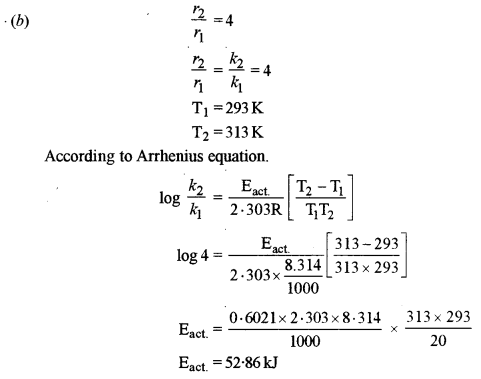Question 3. 
(a) How do antiseptics differ from disinfectants?
(b) State the role of the following chemicals in the food industry:
(i) Sodium benzoate
(ii) Aspartame
(a) Antiseptics: These are the chemical substances which prevent the growth of micro-organisms and may even kill them and are safe to be applied to living tissues. Examples: furacin, tobramycin etc.

Disinfectants: These are the chemical substances which kill micro-organisms but are not safe to be applied to living tissues but are used for non-living substances such as drains, toilet etc. For example 1% solution of phenol.

(b) (i) Food preservative
(ii) Artificial sweetener

Question 4. 
An aromatic organic compound [A] on heating with NH3 and Cu2O at high pressure gives [B], The compound [B] on treatment with an ice-cold solution of NaNO2 and HCl gives [C], which on heating with Cu/HCl gives compound [A] again. Identify the compounds [A], [B] and [C]. Write the name of the reaction for the conversion of [B] to [C].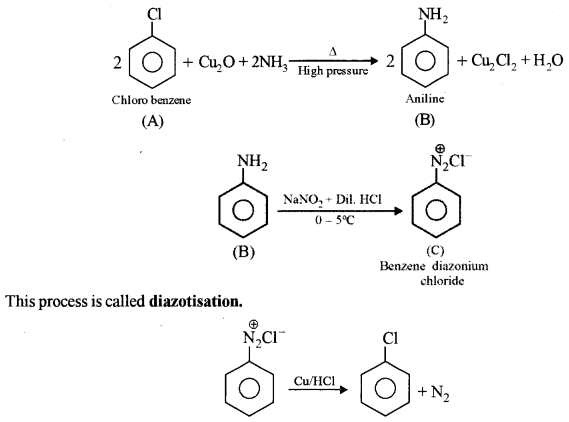A – Chlorobenzene
B – Benzenamine or Aniline
C – Benzene diazonium chloride

Question 5. 
Write the names of the monomers for each of the following polymers:
(a) Bakelite
(b) Nylon – 2 – Nylon – 6
(a) Bakelite
Monomers: Phenol and formaldehyde
(b) Nylon – 2 – Nylon – 6
Glycine and ∈ – Amino Caproic acid

Question 6. 
Name the purine bases and pyrimidine bases present in RNA and DNA.

 Nucleic acid Purine bases Pyrimidine bases RNA DNA Adenine, Guanine Adenine, Guanine Uracil, Cytosine Thymine, Cytosine

Question 7. 
(a) How will you obtain the following? (Give balanced equation.)
(i) Picric acid from phenol.
(ii) Ethyl chloride from diethyl ether.
Or
(b) How will you obtain the following? (Give balanced equation.)
(i) Anisole from phenol
(ii) Ethyl acetate from ethanol.Question 8. 
40% of a first-order reaction is completed in 50 minutes. How much time will it take for the completion of 80% of this reaction?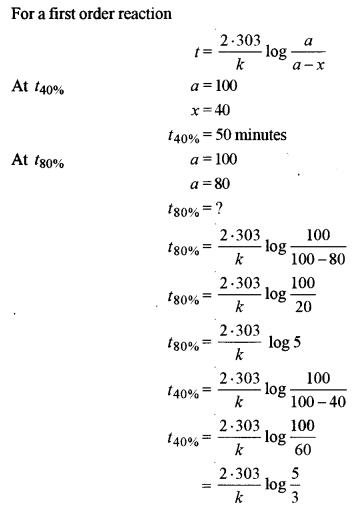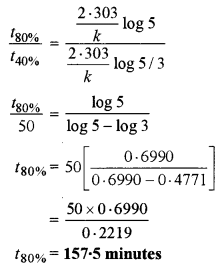Question 9. 
(a) The freezing point of a solution containing 5.85 g of NaCl in 100 g of water is -3.348°C. Calculate Van’t Hoff factor ‘i’ for this solution. What will be the experimental molecular weight of NaCl? (Kf for water = 1.86 K kg mol-1, at. wt. Na = 23, Cl = 35.5)
Or
(b) An aqueous solution containing 12.48 g of barium chloride (BaCl2) is 1000g of water, boils at 100.0832°C. Calculate the degree of dissociation of barium chloride. (Kb for water = 0.52 K kg mol-1, at. wt. Ba = 137, Cl = 35.5)
(a) ΔTf = 3.348°C
WB = 5.85g
WA = 100g
Kf = 1.86 K kg mol-1
Observed mol. mass of NaCl(MB)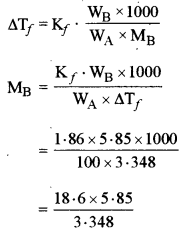Observed mol. mass of NaCl = 32.5 g mol-1
Normal mol. mass of NaCl = 23 + 35.5 = 58.5 g mol-1
Van’t Hoff factor, i = $$\frac{58 \cdot 5}{32 \cdot 5}$$ = 1.8
Or
Kb = 0.52 K kg mol-1
WB = 100 g = 0.01 kg
ΔTb = 100.0832 – 100 = 0.0832°C = 0.0832 K
Molality of BaCl2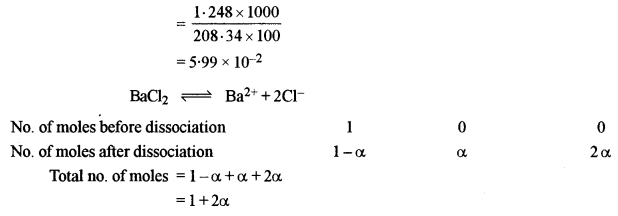Molality after dissociation = 5.99 × 10-2(1 + 2α)
ΔTb = Kb × m
0.0832 = 0.52 × 5.99 × 10-2 (1 + 2α)
0.0832 = 0.0312 + 0.0624α
α = 0.833 = 83.3%

Question 10.
Examine the defective crystal given below and answer the question that follows: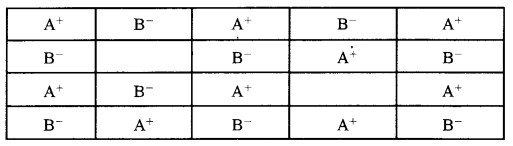State if the above defect is stoichiometric or non-stoichiometric. How does this defect affect the density of the crystal? Also, write the term used for this type of defect.
(i) 1. Stoichiometric defect
2. Density decreases
3. Schottky defect

Question 11.
Give the reason for each of the following: 

1. For ferric hydroxide sol, the coagulating power of phosphate ion is more than chloride ion.
2. Medicines are more effective in their colloidal form.
3. Gelatin is added to ice creams.

1. This is because $$\mathrm{PO}_{4}^{3-}$$ ion has more charge than Cl ion.
2. Phosphate ion $$\mathrm{PO}_{4}^{3-}$$
3. To stabilise ice creams.

Question 12.
(a) For the complex ion [Fe(CN)6]3- state: 
(i) the type of hybridisation.
(ii) the magnetic behaviour.
(iii) the oxidation number of the central metal atom.
(b) Write the IUPAC name of [Co(en)2Cl2]+ ion and draw the structures of its geometrical isomers.
(a) (i) d2sp3
(ii) Paramagnetic due to one unpaired electron
(iii) +3
(b) dichlorobis (ethylene diamine) cobalt (III) ion.Question 13.
(a) Explain why: 
(i) Mn2+ is more stable than Fe2+ towards oxidation to +3 state.
(At. no. of Mn = 25, Fe = 26)
(ii) Transition elements usually form coloured ions.
(iii) Zr and Hf exhibit similar properties.
(At. no. of Zr = 40, Hf = 72)
Or
(b) Complete and balance the following chemical equations:
(i) KMnO4 + KI + H2SO4 → ____ + ____ + ____ + _____
(ii) K2Cr2O7 + H2SO4 + H2S → ____ + _____ + _____ + ____
(iii) KMnO4 + H2SO4 + FeSO4 → _____ + _____ + _____ + ____
(a) (i) This is because Mn2+(3d5) has extra stable electronic configuration but Fe2+(3d6) does not.
(ii) This is due to the presence of impaired electrons in their (n-1) d – orbitals.
(iii) This is due to lanthanide contraction or ineffective shielding but intervening 4f- electrons in Hf.
Or
(b) (i) 2KMnO4 + 10KI + 8H2SO4 → 6K2SO4 + 2MnSO4 + 8H2O + 5I2
(ii) K2Cr2O7 + 4H2SO4 + 3H2S → K2SO4 + Cr2(SO4)3 + 7H2O + 3S
(iii) 2KMnO4 + 8H2SO4 + 10FeSO4 → K2SO4 + 2MnSO4 + 5Fe2(SO4)3 + 8H2O

Question 14.
(a) Arrange the following in the increasing order of their basic strength: 
C2H5NH2, C6H5NH2, (C2H5)2NH
(b) Give a balanced chemical equation to convert methyl cyanide to ethyl alcohol.
(c) What happens when benzene diazonium chloride reacts with phenol in weak alkaline medium? (Give balanced equation).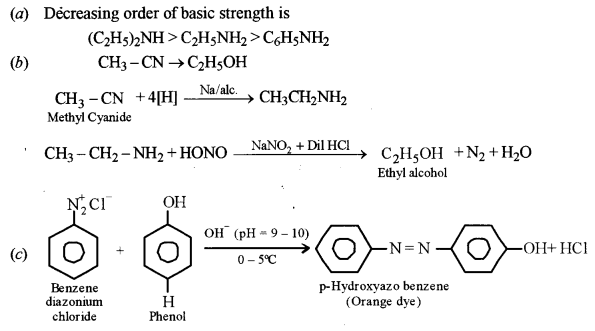Question 15.
Name the sulphide ore of Copper. Describe how pure copper is extracted from this ore. 
The sulphide ore of copper is copper pyrite (CuFeS2). The extraction of pine copper from copper pyrite ore involves the following steps.
1. Crushing and grinding
2. Concentration by the froth-floatation process to remove gangue particles.
3. Roasting in the presence of free supply of air or O2 during roasting the reactions taking place are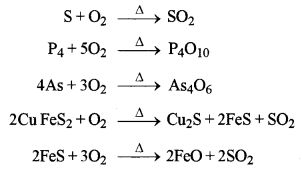The roasted ore mainly contains a number of Cu2S, FeS and FeO.

4. Smelting: The roasted ore is mixed with powdered coke and sand and is heated strongly in a blast furnace.The mixture thus obtained contains Cu2S and some FeS. It is called the molten matte.

5. Bessemerisation: In Bessemer converter, a hot blast of air mixed with sand is blown through molten matte. The reactions taking place are: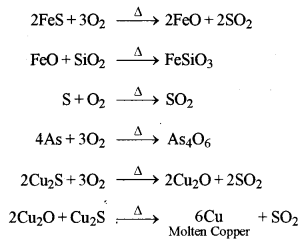On cooling molten copper, we get blister copper.

6. Refining of Copper: The copper obtained above is refined by poling and then by electrolyte refining containing acidified copper sulphate solution as an electrolyte.

Question 16.
(a) (i) Calculate the emf and ΔG° for the cell reaction at 25°C: 
$$\begin{array}{cl}{\mathrm{Zn}(s) | \mathrm{Zn}_{(a q)}^{2+} \|} & {\mathrm{Cd}_{(a q)}^{2+} | \mathrm{Cd}_{(s)}} \\ {(0 \cdot 1 \mathrm{M})} & {(0 \cdot 01 \mathrm{M})}\end{array}$$
Given E° Zn2+/Zn = – 0-763 and E°Cd2+/Cd = -0.403
(ii) Define the following terms:
(1) Equivalent conductivity
(2) Corrosion of metals
Or
(b) (i) The specific conductivity of a solution containing 5 g of an hydrous BaCl2 (mol. wt. = 208) in 1000 cm3 of a solution is found to be 0.0058 ohm-1 cm-1. Calculate the molar and equivalent conductivity of the solution.
(ii) What is an electrochemical series? How is it useful in predicting whether a metal can liberate hydrogen from acid or not?
(a) (i) The given electrochemical cell is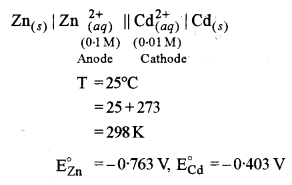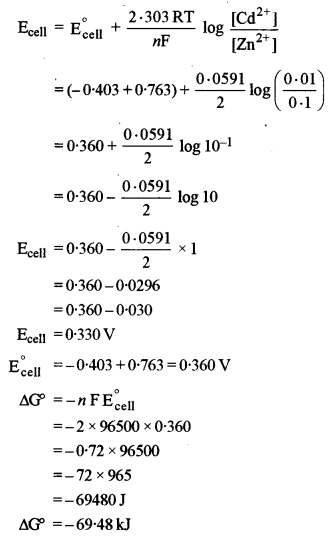(ii) (1) Equivalent Conductivity: It is the conducting power of all the ions present in a solution containing one gram equivalent of the electrolyte.
(2) Corrosion of Metals: It is the process involving the conversion of the metal surface into undesirable compounds on exposure to atmospheric conditions i.e. moisture and air. It is also called weeping of metals.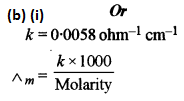(ii) It is a series or table in which the various electrodes are arranged in the increasing order of standard reduction potential values. With the help of the electrochemical series, we can predict whether a given metal can liberate hydrogen from acid or not. The metals which lie above hydrogen in the electrochemical series i.e. having negative standard reduction potential values can liberate hydrogen from dilute acids whereas metals which lie below hydrogen in the electrochemical series i.e., have positive standard reduction potential values cannot liberate hydrogen from dilute acids.

Question 17.
(a) (i) Explain why: 
(1) Nitrogen does not form pentahalides.
(2) Helium is used for filling weather balloons.
(3) ICl is more reactive than I2.
(ii) Draw the structures of the following:
(1) HClO4
(2) H3PO3
Or
(b) (i) Explain why:
(1) Mercury loses its meniscus in contact with ozone.
(2) Halogens are coloured and the colour deepens on moving down in the group from fluorine to iodine.
(3) Hydride of sulphur is a gas while hydride of oxygen is a liquid.
(ii) Complete and balance the following reactions:
(1) NaCl + MnO2 + H2SO4 → _____ + _____ + ______ + _____
(2) KMnO4 + SO2 + H2O → _____ + ______ + ______ + ______
(a) (i) (1) Nitrogen does not form pentahalides because it has no vacant d-orbitals in its valence shell.
(2) This is because it is lighter than air.
(3) This is because ICl is polar and has lower bond energy than I2 (non-polar)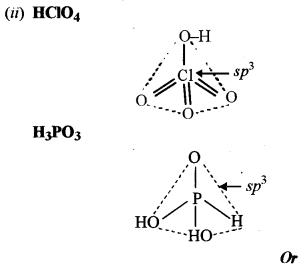(b) (i) (1) This is due to the formation of mercurous oxide (Hg2O) which dissolves in mercury.
(2) This is because halogen molecules (X2) absorb light in the visible region and transmit complementary colour. This is because on moving from fluorine to iodine ionisation enthalpy decreases.
(3) This is because in H2S there are weak Van der Waals forces of attraction but in H2O there are strong intermolecular H-bonds.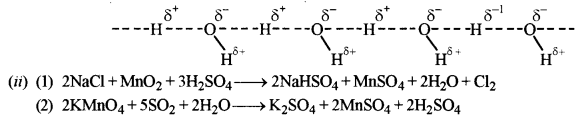Question 18.
(a) (i) Give balanced equations for the following reactions: 
(1) Benzaldehyde reacts with hydrazine.
(2) Acetic acid reacts with phosphorous pentachloride.
(3) Acetone reacts with sodium bisulphite.
(ii) Give one chemical test each to distinguish between the following pairs of compounds:
(1) Ethanol and acetic acid
(2) Acetaldehyde and benzaldehyde
Or
(b) (i) Write chemical equations to illustrate the following name reactions:
(1) Clemmensen’s reduction
(2) Rosenmund’s reduction
(3) HVZ reaction
(iii) Explain why:
(1) Acetaldehyde undergoes aldol condensation but formaldehyde does not.
(2) Acetic acid is a weaker acid as compared to formic acid.